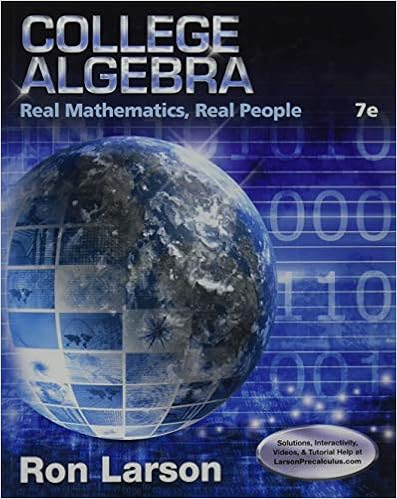old-sample-questions - Topics for the 1st Midterm Math 222...

• Notes
• 6

This preview shows page 1 - 2 out of 6 pages.

We have textbook solutions for you!
The document you are viewing contains questions related to this textbook.The document you are viewing contains questions related to this textbook.
Chapter 3 / Exercise 4
College Algebra: Real Mathematics, Real People
LarsonExpert Verified
Topics for the 1st Midterm Math 222, “Spring” 2009 Topics covered on this exam The first midterm will be on the material covered in sections 1–16 of the lecture notes. Integration. You should be able to use the following methods to compute integrals. Substitution Integration by parts Reduction formulas: know how to use them if you are given one, and know how to derive one. Partial fractions. Taylor polynomials. You should know how to compute T a f ( x ) (i.e. give a formula for the coefficient of x n ) by computing f ( n ) ( a ). Use partial fractions to compute Taylor series of simple rational functions. Use Lagrange’s formula for the remainder to estimate the error term R n f ( x ) if x and n are explicitly given, or “for | x | ≤ 0 . 2”. Understand the “little-oh” notation. Use a combination of “little-oh”, “standard Taylor series”, algebra of Taylor polynomials (in- cluding “ T n [ f 0 ( x )] = derivative of T n +1 [ f ( x )]”) to efficiently compute Taylor polynomials. Theory Questions The following questions may not necessarily appear on the midterm, but answering them will help you find gaps in your understanding of the material. (1) State the Fundamental Theorem of Calculus. (2) The integral R 1 0 4( x + a ) 3 d x contains two variables, x and a . Which of these is a dummy variable and why? (3) State and derive the formula for integration by parts. (4) Explain to a friend what the partial fraction expansion of a rational function looks like and how you find it. (5) What is the Taylor polynomial of degree n of a function f ( x ) at the point a ? (6) State the Geometric Sum Formula. (7) What is the difference between a Taylor and a Maclaurin polynomial? (8) What is the remainder term in a Taylor polynomial? (9) State Lagrange’s formula for the remainder term in a Taylor polynomial. (10) What does it mean for a function f ( x ) to be o ( x n ) as x 0? Sample Problems for the 1st Midterm These are problems from 222 midterms that were given in the past, and they are relevant to the coming first midterm. Other old exams are available on the web, but be warned that the syllabus changes every year, so that problems which were on the 1st midterm 2 years ago may show up on this year’s 2nd midterm, and vice versa . Don’t prepare for last year’s midterm!
We have textbook solutions for you!
The document you are viewing contains questions related to this textbook.The document you are viewing contains questions related to this textbook.
Chapter 3 / Exercise 4
College Algebra: Real Mathematics, Real People
LarsonExpert Verified
•••